# Tree Traversal - inorder, preorder and postorder

Traversing a tree means visiting every node in the tree. You might for instance want to add all the values in the tree or find the largest one. For all these operations, you will need to visit each node of the tree.

Linear data structures like arrays, stacks, queues and linked list have only one way to read the data. But a hierarchical data structure like a tree can be traversed in different ways.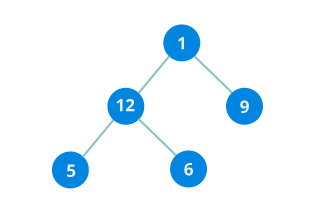Let's think about how we can read the elements of the tree in the image shown above.

Starting from top, Left to right

``1 -> 12 -> 9 -> 5 -> 6``

Starting from bottom, Left to right

``5 -> 6 -> 12 -> 9 -> 1``

Although this process is somewhat easy, it doesn't respect the hierarchy of the tree, only the depth of the nodes.

Instead, we use traversal methods that take into account the basic structure of a tree i.e.

``````struct node {
int data;
struct node* left;
struct node* right;
}``````

The struct node pointed to by left and right might have other left and right children so we should think of them as sub-trees instead of sub-nodes.

According to this structure, every tree is a combination of

• A node carrying data
• Two subtrees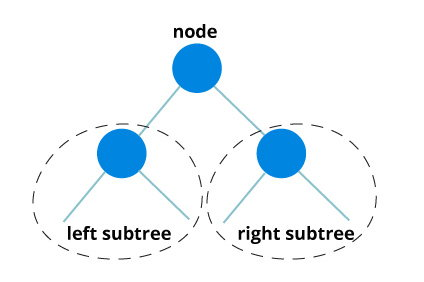Remember that our goal is to visit each node, so we need to visit all the nodes in the subtree, visit the root node and visit all the nodes in the right subtree as well.

Depending on the order in which we do this, there can be three types of traversal.

## Inorder traversal

1. First, visit all the nodes in the left subtree
2. Then the root node
3. Visit all the nodes in the right subtree
``````inorder(root->left)
display(root->data)
inorder(root->right)``````

## Preorder traversal

1. Visit root node
2. Visit all the nodes in the left subtree
3. Visit all the nodes in the right subtree
``````display(root->data)
preorder(root->left)
preorder(root->right)``````

## Postorder traversal

1. visit all the nodes in the left subtree
2. visit the root node
3. visit all the nodes in the right subtree
``````postorder(root->left)
postorder(root->right)
display(root->data)``````

Let's visualize in-order traversal. We start from the root node.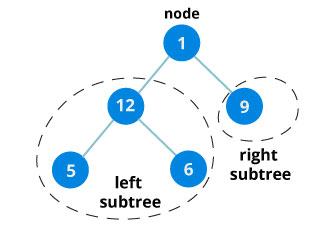We traverse the left subtree first. We also need to remember to visit the root node and the right subtree when this tree is done.

Let's put all this in a stack so that we remember.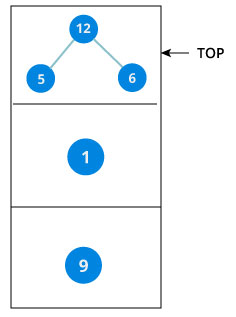Now we traverse to the subtree pointed on the TOP of the stack.

Again, we follow the same rule of inorder

`Left subtree -> root -> right subtree`

After traversing the left subtree, we are left with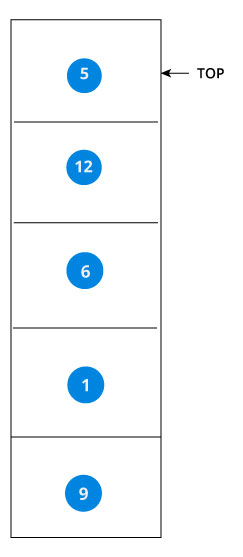Since the node "5" doesn't have any subtrees, we print it directly. After that we print its parent "12" and then the right child "6".

Putting everything on a stack was helpful because now that the left-subtree of the root node has been traversed, we can print it and go to the right subtree.

After going through all the elements, we get the inorder traversal as

`5 -> 12 -> 6 -> 1 -> 9`

We don't have to create the stack ourselves because recursion maintains the correct order for us.

The complete code for inorder, preorder and postorder in C programming language is posted below:

``````#include <stdio.h>
#include <stdlib.h>

struct node {
int data;
struct node* left;
struct node* right;
};

void inorder(struct node* root){
if(root == NULL) return;
inorder(root->left);
printf("%d ->", root->data);
inorder(root->right);
}

void preorder(struct node* root){
if(root == NULL) return;
printf("%d ->", root->data);
preorder(root->left);
preorder(root->right);
}

void postorder(struct node* root) {
if(root == NULL) return;
postorder(root->left);
postorder(root->right);
printf("%d ->", root->data);
}

struct node* createNode(value){
struct node* newNode = malloc(sizeof(struct node));
newNode->data = value;
newNode->left = NULL;
newNode->right = NULL;

return newNode;
}

struct node* insertLeft(struct node *root, int value) {
root->left = createNode(value);
return root->left;
}

struct node* insertRight(struct node *root, int value){
root->right = createNode(value);
return root->right;
}

int main(){
struct node* root = createNode(1);
insertLeft(root, 12);
insertRight(root, 9);

insertLeft(root->left, 5);
insertRight(root->left, 6);

printf("Inorder traversal \n");
inorder(root);

printf("\nPreorder traversal \n");
preorder(root);

printf("\nPostorder traversal \n");
postorder(root);
}``````

The output of the code will be

```Inorder traversal
5 ->12 ->6 ->1 ->9 ->
Preorder traversal
1 ->12 ->5 ->6 ->9 ->
Postorder traversal
5 ->6 ->12 ->9 ->1 ->```